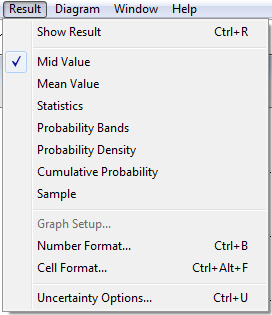The Result menu allows to view result tables and graphs, and to configure how deterministic and non-deterministic (uncertain) values are displayed there.

The functionalities of the individual items of the Result menu are explained in the table below.

Menu item DescriptionShow Result Opens a Result window for the selected object. See Result window. Mid Value Displays the mid or deterministic value. See Uncertainty view of a result. Mean Value Displays the mean of the uncertain value. See Uncertainty view of a result. Statistics Displays statistics of the uncertain value in a table as set in the Uncertainty Setup dialog. See Uncertainty view of a result. Probability Bands Shows probability bands (percentiles) as set in the Uncertainty Setup dialog. See Uncertainty view of a result. Probability Density Displays a probability density graph for an uncertain value. For a discrete probability distribution, Probability Mass replaces this command. See Uncertainty view of a result. Cumulative Probability Displays a cumulative probability graph representing the probability that a variable’s value is less than or equal to each possible (uncertain) value. See Uncertainty view of a result. Exceedance Probability Displays an exceedance probability graph representing the probability that a variable’s value is greater than or equal to each possible (uncertain) value. See Uncertainty view of a result. This option only appears in Analytica 5.4 or after. Sample Displays a table of the values determined for each uncertainty sample iteration. See Uncertainty view of a result. Graph Setup Displays a dialog to specify the graphing tool, graph frame, and graph style. See Graphing roles. Number Format Displays a dialog to set the number format for displays of results. See Number formats. Cell Format... (New to Analytica 5.0). Displays a dialog to change the cell-level appearance or behavior of table cells, including cell colors, borders, fonts, alignment, entry type and number formats. Uncertainty Options Displays a dialog to specify the uncertainty sample size and sampling method and to set options for statistics, probability bands, probability density, and cumulative probability. See Uncertainty Setup dialog.